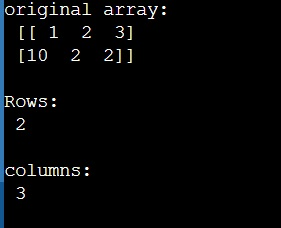# NumPy: How to find total rows in a 2D array and total column in a 1D array?

Learn, how to find total rows in a 2D array and total column in a 1D array in Python NumPy?
Submitted by Pranit Sharma, on March 22, 2023

## Finding total rows in a 2D array and total column in a 1D array

Suppose that we are given a 2D numpy array and we need to find the total number of rows in this 2D array.

If we consider a single row of a numpy array, it is nothing but a 1D array and we need to find the total number of columns in this 1D array too.

However, the total number of elements in a row is the total number of columns in that row/array. To know the total number of rows and columns in a 2D array, we can use the arr.shape() function which will return a tuple of dimensions of an array where the first dimension is the number of rows and another dimension is the number of columns.

Let us understand with the help of an example,

## Python code to find total rows in a 2D array and total column in a 1D array

```# Import numpy
import numpy as np

# Creating a numpy array
arr = np.array([[1,2,3],[10,2,2]])

# Display original array
print("original array:\n",arr,"\n")

# Getting rows and columns
rows, cols = arr.shape

# Display result
print("Rows:\n",rows,"\n")
print("columns:\n",cols)
```

### Output